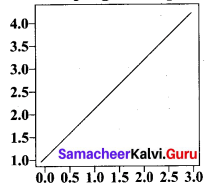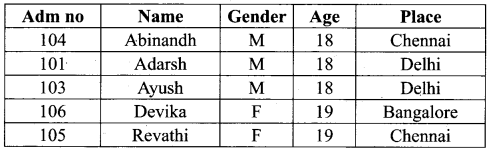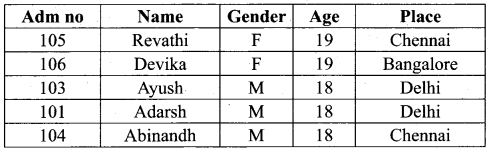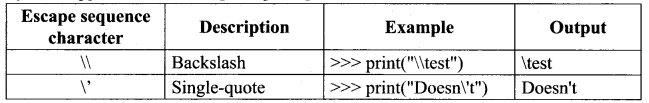# Tamil Nadu 12th Computer Science Model Question Paper 1 English Medium

Students can Download Tamil Nadu 12th Computer Science Model Question Paper 1 English Medium Pdf, Tamil Nadu 12th Computer Science Model Question Papers helps you to revise the complete Tamilnadu State Board New Syllabus and score more marks in your examinations.

## TN State Board 12th Computer Science Model Question Paper 1 English Medium

General Instructions:

1. The question paper comprises of four parts.
2. You are to attempt all the parts. An internal choice of questions is provided wherever applicable.
3. All questions of Part I, II, III and IV are to be attempted separately.
4. Question numbers 1 to 15 in Part I are Multiple Choice Questions of one mark each.
These are to be answered by choosing the most suitable answer from the given four alternatives and writing the option code and the corresponding answer
5. Question numbers 16 to 24 in Part II are two-mark questions. These are to be answered in about one or two sentences.
6. Question numbers 25 to 33 in Part III are three-mark questions. These are to be answered in about three to five short sentences.
7. Question numbers 34 to 38 in Part IV are five-mark questions. These are to be answered in detail Draw diagrams wherever necessary.

Time: 3 Hours
Maximum Marks: 70

Part – I

Choose the correct answer. Answer all the questions: [15 × 1 = 15]

Question 1.
The file extension to save excel files are _______.
(a) xls or xlsx
(b) XL
(c) exe or XL
(d) XL or XLX
(a) xls or xlsx

Question 2.
Python has a collector to clean up unreferenced object.
(a) CSV
(b) Diet
(c) garbage
(d) close
(c) garbage

Question 3.
How many types of DML are there?
(a) 1
(b) 2
(c) 3
(d) 4
(b) 2

Question 4.
To open the file updating data, click _______.
(a) a
(b) b
(c) t
(d) +
(d) +Question 5.
g++ is a program that calls GNU C compiler called _______.
(a) GCC
(b) CCC
(c) GGC
(d) GGG
(a) GCC

Question 6.
________ are used for interfacing with C programs.
(a) C types
(b) cython
(c) M in GW
(d) Boost python
(a) C types

Question 7.
Which function is used to print the data in dictionary format without order?
(a) dic()
(b) dict()
(c) dictionary
(d) print
(b) dict()

Question 8.
________ is a list containing another list as an element.
(a) N list
(b) Nested list
(c) List
(b) Nested list

Question 9.
In python, _______ function is used to accept data as input at run time.
(a) accept
(b) int
(c) input
(d) in
(c) inputQuestion 10.
Which one of the following is the exponential operator?
(a) *
(b) **
(c) ++
(d) //
(b) **

Question 11.
A ______ is a part of a program.
(a) code
(b) module
(c) flow chart
(d) system software
(b) module

Question 12.
The arrangement of private instance variables and public methods ensures the principle of _______.
(a) security
(b) data encapsulation
(c) class
(d) inheritance
(b) data encapsulation

Question 13.
How many operands are there in ternary operator?
(a)0
(b) 1
(c)2
(d) 3
(d) 3

Question 14.
Pow (2, -3) gives ________.
(a) 8
(b) -8
(c) 0.125
(d) 1
(c) 0.125Question 15.
______ assign values to the tables specified in the bar chart.
(a) usage
(b) label
(c) values
(d) =
(a) usage

Part – II

Answer any six questions. Question No. 21 is compulsory. [6 x 2 = 12]

Question 16.
Differentiate Interface and Implementation.
Interface:
Interface just defines what an object can do, but won’t actually do it.

Implementation:
Implementation carries out the instructions defined in the interface.

Question 17.
Give example for valid Identifiers.
Sum, total marks, regno, num 1.Question 18.
Define SQL.
The Structured Query Language (SQL) is a standard programming language to access and manipulate databases. SQL allows the user to create, retrieve, alter, and transfer information
among databases.

Question 19.
Give example for open function.
>>> f = open(“sample.txt”) # open file in current directory and f is file object
>>> f = open(‘c:\\pyprg\\ch 13sample5.csv’) # specifying full path

Question 20.
Constructor is the special function that is automatically executed when an object of a class is created. In Python, there is a special function called “init” which act as a Constructor. It must begin and end with double underscore. This function will act as an ordinary function; but only difference is, it is executed automatically when the object is created. This constructor function f can be defined with or without arguments. This method is used to initialize the class variables.

Question 21.
Write a program to print the following output.Example
import matplotlib.pyplot as plt
plt.plot([l ,2,3,4])
plt.show()
Output
This window is a matplotlib window, which allows you to see your graph. You can hover the graph and see the coordinates in the bottom right.

Question 22.
Which punctuation should be used in the blank?
if <condition>_
statements-block 1
else:
statements-block 2
(a) ;
(b) :
(c) ::
(d) !
(6) :Question 23.
Define Required Arguments.
“Required Arguments” are the arguments passed to a function in correct positional order. Here, the number of arguments in the function call should match exactly with the function definition. You need atleast one parameter to prevent syntax errors to get the required output.

Question 24.
Write note on Assignment operators.
In Python, = is a simple assignment operator to assign values to variable. Let a = 5 and b = 10 assigns the value 5 to a and 10 to b these two assignment statement can also be given as a,b=5,10 that assigns the value 5 and 10 on the right to the variables a and b respectively. There are various compound operators in Python like +=, -=, *=, /=, %=, **= and //= are also available.

Part – III

Answer any six questions. Question No. 29 is compulsory. [6 × 3 = 18]

Question 25.
Write note on Asymptotic Notations. Classify them.
Asymptotic Notations are languages that uses meaningful statements about time and space complexity. The following three asymptotic notations are mostly used to represent time complexity of algorithms:
(i) Big O
Big O is often used to describe the worst-case of an algorithm.

(ii) Rig Ω
Big Omega is the reverse Big O, if Bi O is used to describe the upper bound (worst – case) of a asymptotic function, Big Omega is used to describe the lower bound (best-case).

(iii) Big Θ
When an algorithm has a complexity with lower bound = upper bound, say that an algorithm has a complexity O (n log n) and Ω (n log n), it’s actually has the complexity Θ (n log n), which means the running time of that algorithm always falls in n log n in the best-case and worst-case.Question 26.
Write note on CSV files.
CSV File cannot store charts or graphs. It stores data but does not contain formatting, formulas, macros, etc.
A CSV file is also known as a Flat File. Files in the CSV format can be imported to and exported from programs that store data in tables, such as Microsoft Excel or Open Office Calc.

Question 27.
Write note on where clause in SQL.
The WHERE clause is used to filter the records. It helps to extract only those records which satisfy a given condition. For example in the student table, to display the list of students of age 18 and above in alphabetical order of their names, the command is given as below: SELECT * FROM Student WHERE Age>=18 ORDER BY Name;To display the list of students in the descending order of names of those students of age 18 and above the command is given as :
SELECT * FROM Student WHERE Age>= 18 ORDER BY Name DESC;Question 28.
Give an example and syntax for group by clause.
The syntax for the GROUP BY clause is
SELECT <column-names> FROM <table-name> GROUP BY <column-name>HAVING condition];
To apply the above command on the student table :
SELECT Gender FROM Student GROUP BY Gender;
The following command will give the below given result:
Gender
M
F

The point to be noted is that only two results have been returned. This is because we only have two gender types ‘Male’ and ‘Female’. The GROUP BY clause grouped all the ‘M’ students together and returned only a single row for it. It did the same with the ‘F’ students. For example to count the number of male and female students in the student table, the following command is given :

SELECT Gender, count(*) FROM Student GROUP BY Gender;

 Gender Count* M 5 F 3Question 29.
Illustrate with an example program to change global variable from inside a function using global keyword.
x = 0 # global variable
global x
x = x + 5 # increment by 2
print (“Inside add() function x value is :”,x)
print (“In main x value is :”,x)
Output:
Inside add() function x value is : 5
In main x value is : 5

Question 30.
Write note on Lambda functions.

• Lambda function is mostly used for creating small and one-time anonymous function.
• Lambda functions are mainly used in combination with the functions like filter(), map() and reduce().

Question 31.
Give any 3 applications of scripting language.

1. To automate certain tasks in a program.
2. Extracting information from a data set.
3. Less code intensive as compared to traditional programming language.

Question 32.
Give program to join two tuples
# Program to join two tuples
Tup 1 =(2,4,6,8,10)
Tup2 = (1,3,5,7,9)
Tup3 = Tup1 + Tup2
print(Tup3)
Output
(2, 4, 6, 8, 10, 1, 3, 5, 7, 9)

Question 33.
Find output:
for x in range (2,11,2) :
Print (x)
Output:
2 4 6 8 10

Part – IV

Answer all the following questions. [5 × 5 = 25]

Question 34.
(a) Compare CSV files with XLS file.
Excel:

• Excel is a binary file that holds information about all the worksheets in a file, including both content and formatting.
• XLS files can only be read by applications that have been especially written to read their format, and can only be written in the same way.
• Excel is a spreadsheet that saves files into its own proprietary format viz. xls or xlsx.
• Excel consumes more memory while importing data.

CSV:

• CSV format is a plain text format with a series of values separated by commas.
• CSV can be opened with any text editor in Windows like notepad, MS Excel, OpenOffice, etc.
• CSV is a format for saving tabular information into a delimited text file with extension .csv
• Importing CSV files can be much faster, and it also consumes less memory.

[OR]

(b) Explain any 5 important features of python over C++.

• Python uses Automatic Garbage Collection whereas C++ does not.
• C++ is a statically typed language, while Python is a dynamically typed language.
• Python runs through an interpreter, while C++ is pre-compiled.
• Python code tends to be 5 to 10 times shorter than that written in C++.
• In Python, there is no need to declare types explicitly where as it should be done in C++
• In Python, a function may accept an argument of any type, and return multiple values without any kind of declaration beforehand. Whereas in C++ return statement can return only one value.Question 35.
(a) Write a program to update a change range of values.
MyList = [1, 3, 5, 7, 9]
print (“List Odd numbers… “)
for x in MyList:
print (x)
MyList[0:5] = 2,4,6,8,10
print (“List Even numbers… “)
for y in MyList:
print (y)

Output
List Odd numbers…
1
3
5
7
9
List Even numbers…
2
4
6
8
10

[OR]

(b) Explain list and range ( ) functions in python with sample program.
The range( ) is a function used to generate a series of values in Python. Using range( ) function, you can create list with series of values. The range() function has three arguments.
Syntax of range ( ) function:
range (start value, end value, step value)
where,

• start value – beginning value of series. Zero is the default beginning value.
• end value – upper limit of series. Python takes the ending value as upper limit – 1.
• step value – It is an optional argument, which is used to generate different interval of values.

Example : Generating whole numbers up to 10
for x in range (1, 11):
print(x)

Output
1
2
3
4
5
6
7
8
9
10

Question 36.
(a) Find output:
T = (12, 78, 91, “Tamil”, “Telugu”, 3.14, 69.48)
1. Print (T)
2. Print ( T [2:5] )
3. Print ( T[:5] )
4. Print ( T[4:] )
5. Print ( T [:])
1. (12, 78, 91, “Tamil”, “Telugu”, 3.14, 69.48)
2. (91, “Tamil”, “Telugu”)
3. (12, 78, 91, “Tamil”, “Telugu”)
4. (“Telugu”, 3.14, 69.48)
5. (12, 78, 91, “Tamil”, “Telugu”, 3.14, 69.48)

[OR]

(b) Find Output:
T = (2,4,6, 8,10)
Print (T)
del T
Print (T)
(2, 4, 6, 8, 10)
Name Error: name “T” not defined.Question 37.
(a) Differentiate Algorithm with program.
Algorithm:

• Algorithm helps to solve a given problem logically and it can be contrasted with the program.
• Algorithm can be categorized based on their implementation methods, design techniques etc.
• There is no specific rules for algorithm writing but some guidelines should be followed.
• Algorithm resembles a pseudo code which can be implemented in any language.

Program:

• Program is an expression of algorithm in a programming language.
• Algorithm can be implemented by structured or object oriented programming approach.
• Program should be written for the selected language with specific syntax.
• Program is more specific to a programming language.

[OR]

(b) Explain the various characteristics of an Algorithm.

• Input: Zero or more quantities to be supplied.
• Output: At least one quantity is produced.
• Finiteness: Algorithms must terminate after finite number of steps.
• Definiteness: All operations should be well defined. For example operations involving division by zero or taking square root for negative number are unacceptable.
• Effectiveness: Every instruction must be carried out effectively.
• Correctness: The algorithms should be error free.
• Simplicity: Easy to implement.
• Unambiguous: Algorithm should be clear and unambiguous. Each of its steps and their inputs/outputs should be clear and must lead to only one meaning.
• Feasibility: Should be feasible with the available resources.
• Portable: An algorithm should be generic, independent of any programming language or an operating system able to handle all range of inputs.
• Independent: An algorithm should have step-by-step directions, which should be independent of any programming code.Question 38.
(a) Explain literals in python.
Literal is a raw data given in a variable or constant. In Python, there are various types of literals.
(i) Numeric (ii) String (iii) Boolean

(i) Numeric Literals: Numeric Literals consists of digits and are immutable (unchangeable).
Numeric literals can belong to 3 different numerical types Integer, Float and Complex.
To demonstrate Numeric literals
# Program to demonstrate Numeric Literals
a = 0b 1010 #Binary Literals
b = 100 #Decimal Literal
c = 0o310 #Octal Literal
print (“Integer Literals :”,a,b,c,d)
#Float Literal
float_1 = 10.5
float_2 = 1.5e2
print (“Float Literals :”,float_l,float_2)
#Complex Literal
x = 1 + 3.14 j
print (“Complex Literals :”, x)
Print (“x = “, x , “Imaginary part of x = “, x.imag, “Real part of x = “, x.real)
#End of the Program

Output:
Integer Literals : 10 100 200 300
Float Literals : 10.5 150.0
Complex Literals :
x = (1.3.14) Imaginary part of x = 3.14 Real part of 9 x = 1.0

(ii) String Literals: In Python a string literal is a sequence of characters surrounded by quotes. Python supports single, double and triple quotes for a string. A character literal is a single character surrounded by single or double quotes. The value with triple-quote is used to give multi line string literal.

(iii) Boolean Literals: A Boolean literal can have any of the two values: True or False.

(iv) Escape Sequences: In Python strings, the backslash “\” is a special character, also called the “escape” character. It is used in representing certain whitespace characters: “\t” is a tab, “\n” is a newline, and “\r” is a carriage return. For example to print the message “It’s raining”, the Python command is
>>> print (“It\’s rainning”)
It’s rainning
Python supports the following escape sequence characters.[OR]

(b) Explain Recursive functions in python.
When a function calls itself is known as recursion. Recursion works like loop but sometimes it makes more sense to use recursion than loop. You can convert any loop to recursion.

A recursive function calls itself. Imagine a process would iterate indefinitely if not stopped by some condition! Such a process is known as infinite iteration. The condition that is applied in any recursive function is known as base condition. A base condition is must in every recursive function otherwise it will continue to execute like an infinite loop.

Overview of how recursive function works
(i) Recursive function is called by some external code.
(ii) If the base condition is met then the program gives meaningful output and exits.
(iii) Otherwise, function does some required processing and then calls itself to continue recursion. .

Example:
def fact(n):
if n == 0:
return 1
else:
return n * fact (n-1)
print (fact (0))
print (fact (5))
Output:
1
120